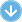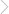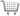88 Pages## Walker Maths 3.14 Probability Distributions Workbook 2nd Edition

### By Charlotte Walker, Victoria Walker | Copyright Year:2020 | ISBN-13: 9780170446938

Published:25/10/2019Walker Maths is a series of single standard workbooks containing high-quality, up to date material at NCEA Mathematics levels 1, 2 and 3.

The well-designed, write-on workbooks contain teaching material, including relevant formulae, and ample practice exercises along with sample tasks and questions. The workbooks reflect the content and style of the new standards, and allow teachers total flexibility in course design for students at all levels.

As a single standard series, Walker Maths offers Maths department the ability to buy titles all at once, or throughout the year as required. Digital Teacher Resources are also available for all standards, please contact your Sales Representative for more details.

### CONTENTS

CONTENTS

Formulae
Glossary
What are probability distributions?
Discrete vs continuous random variables
The language of probability
True probability, experimental probability and theoretical (model) probability

Discrete random variables
1. General distributions
Describing distributions
Calculating the mean, standard deviation and variance of distributions
Linear combinations of a discrete random variable: expectation algebra
Linear combinations of several discrete distributions: expectation algebra
2. The Poisson distribution
Applications of the Poisson distribution
Inverse Poisson problems
3. The binomial distribution
Inverse binomial problems

Continuous random variables
1. The normal distribution
Sketching normal distributions
The standard normal distribution
Calculating probabilities
Inverse normal distribution
Mixed normal distribution problems
Continuity correction
2. The rectangular (continuous uniform) distribution
3. The triangular distribution

Practice questions
Tables
Binomial distribution
Poisson distribution
Normal distribution

Mix and match activity